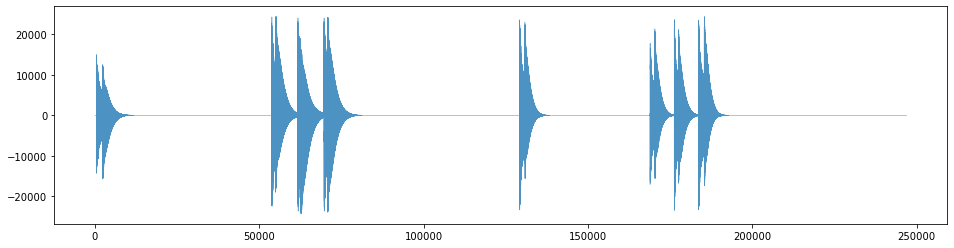## Goal¶

This post aims to introduce how to load wave audio data as an array.

Reference

## Libraries¶

In :
import pandas as pd
import numpy as np
from scipy.io import wavfile
import matplotlib.pyplot as plt
%matplotlib inline


## Load a file as numpy¶

In :
filename = '../data/sound00.wav'
data

Out:
array([0, 0, 0, ..., 0, 0, 0], dtype=int16)

## Visualize audio data¶

In :
plt.figure(figsize=(16, 4))
plt.plot(data, lw=0.5, alpha=0.8);